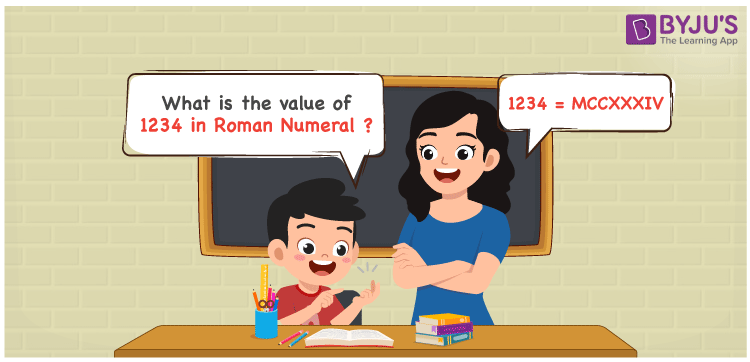# 1234 in Roman Numerals

1234 in Roman Numerals is denoted as MCCXXXIV. The system of expressing numbers using the Roman alphabet is known as Roman Numerals. Students can refer to the article Roman Numerals Conversion to learn the tricks of converting Roman letters into number form in an efficient manner. In this article, let us discuss how to represent 1234 in Roman Numerals.

 Number Roman Numeral 1234 MCCXXXIV

## How to Write 1234 in Roman Numerals?The method used to convert the number 1234 in Roman Numerals is as follows

• Break the number 1234 into the simplest form
• 1234 = 1000 + 100 + 100 + 10 + 10 + 10 + 5 – 1
• Write their respective Roman letter and add/subtract them
• 1234 = M + C + C + X + X + X + V – I = MCCXXXIV
• Thus the value of 1234 in Roman Numerals is MCCXXXIV

## Video Lesson on Roman Numerals## Frequently Asked Questions on 1234 in Roman Numerals

Q1

### What is 1234 in Roman Numerals?

1234 in Roman Numerals is MCCXXXIV.
Q2

### What is the value of 1000 + 234 in Roman Numerals?

1000 + 234 = 1234. The number 1234 in Roman Numerals is MCCXXXIV. Therefore the value of 1000 + 234 in Roman Numerals is MCCXXXIV.
Q3

### What is the difference between 2000 and 1234 in Roman Numerals?

2000 – 1234 = 766. The number 766 in Roman Numerals is DCCLXVI. Thus the difference between 2000 and 1234 in Roman Numerals is DCCLXVI.